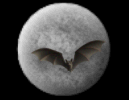God & Science Forum Message Forums: Atm · Astrophotography · Blackholes · Blackholes2 · CCD · Celestron · Domes · Education Eyepieces · Meade · Misc. · God and Science · SETI · Software · UFO · XEphemBe the first pioneers to continue the Astronomy Discussions at our new Astronomy meeting place...The Space and Astronomy Agora The Orbit Is A Function Of Two Things.. Forum List | Follow Ups | Post Message | Back to Thread Topics | In Response ToPosted by Bruce on June 22, 2003 23:45:18 UTC

Energy per unit mass and angular momentum per unit mass

The equation of motion for the Schwarzschild solution is

(dr/dTau)^2 = (E/m)^2 - (1-2M/r)[1+(L/m)^2/r^2]

The mass and angular momentum of the orbiting body. When (dr/dTau)^2 = 0 the orbit is stable. For light it is

dr/dt = (1-2M/r)[1-(1-2M/r)b^2_light/r^2]^1/2

where

b_impact parameter = L/(E^2-m^2)^1/2

and when m>0

b_light = L/E

BTW the local shell

v^2_shell = M/r(1-2M/r) = M/(r-2M)

For R = 3M [the photon sphere]

v^2_shell = 1 [c=1]